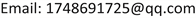The Proof Problem of a Special Positive Definite Matrix

Xiaojing Xu

Shenyang Normal University, Shenyang LiaoningReceived: Mar. 27th, 2021; accepted: Apr. 15th, 2021; published: Apr. 29th, 2021ABSTRACT

Positive definite matrix is a special kind of matrix. As a subclass of symmetric matrix, positive definite matrix has a wide range of applications in mathematical analysis, such as discriminating the extreme value of multivariate functions and judging the monotonicity of functions. It possesses not only the diagonalization property of symmetric matrices, but also the higher property that symmetric matrices do not possess. But in order to use these properties of positive definite matrices, we first have to know that a matrix is positive definite. And you can see that there are a lot of proofs for positive definite matrices that are given in some books or in some tutorial books. You usually solve for the eigenvalues, and then you prove that the eigenvalues are greater than zero. However, in some more complex topics, a large number of proofs of intermediate conclusions are often mixed in. If scholars ignore the proofs of implicit conclusions in the process of thinking, they will fail to complete the whole topic. Therefore, to do this kind of problem often requires us to have a large knowledge reserve. Today we are going to discuss the proof for a special class of matrices, and give a simple proof method.

Keywords:Positive Definite Matrix, Similar, Contract1. 引言

2. 预备知识

1) n阶实对称矩阵A是正定矩阵的充分必要的条件是，存在n阶实可逆矩阵C使得 A = C T C or C C T 。

2) n阶实对称矩阵A是正定矩阵的充分必要的条件是，A的特征值都大于零。

3) 是对称矩阵可正交相似于对角阵，即对于任意一个是对称矩阵A，都存在n阶正交矩阵Q，使得 Q − 1 A Q = Q T A Q 为对角阵。

4) 一个实矩阵是正定的当且仅当它与单位矩阵合同。

1) 若A是实对称矩阵。则存在正交矩阵P，使得 P ′ A P = P − 1 A P = [ λ 1 ⋱ λ n ] 为对角阵。

2) 反对称矩阵的特征值只能是零或者是成对出现的纯虚数。

3. 符号说明

Description of symbols in the matri

4. 正定矩阵的证明的问题4.1. 例1

A B ∈ R n × n ， A B = B A ，则有 ( A B ) = B ′ A ′ = B A ，AB是n阶是对称矩阵。存在正交矩阵P，使得 P − 1 A P = [ λ 1 E 1 ⋱ λ s E s ] ，其中 E i 为 k 阶单位矩阵， λ 1 ⋯ λ s 互不相同。

[ λ 1 E 1 ⋱ λ S E S ] 为对角阵。故在同一个n阶实对称矩阵使得 T − 1 A T = [ a 1 ⋱ a n ] ， T − 1 B T = [ b 1 ⋱ b n ] 。题目已知 A , B 都是正定矩阵，所以有 a i > 0 , b i > 0 ( i = 1 , 2 , ⋯ , n ) 。但有 T − 1 A B T = ( T − 1 A T ) ( T − 1 B T ) = [ a 1 b 1 ⋱ a n b n ] ，因为 a i b i > 0 ( i = 1 , 2 , ⋯ , n ) ，所以AB为正定矩阵。即得证。

A = Q Λ Q T = Q d i a g λ 1 , λ 2 , ⋯ , λ n d i a g λ 1 , λ 2 , ⋯ , λ n Q T = Q d i a g λ 1 , λ 2 , ⋯ , λ n Q T Q d i a g λ 1 , λ 2 , ⋯ , λ n Q T = B 2 .

4.2. 例2

4.3. 小结

5. 应用

6. 总结